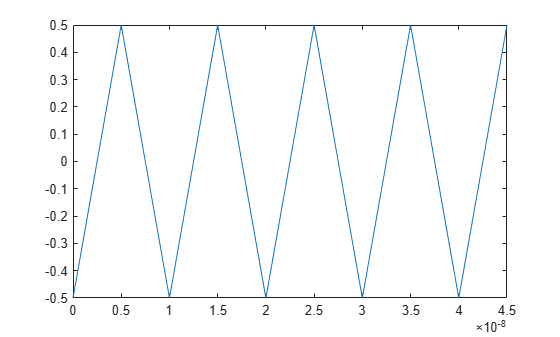# timeDomainSignal2RiseTime

Measure rise time of time domain signal

## Syntax

``Tr = timeDomainSignal2RiseTime(Tin,Vin,RefLevel)``

## Description

example

````Tr = timeDomainSignal2RiseTime(Tin,Vin,RefLevel)` returns the rise time of the time-domain input signal represented by [`Tin`, `Vin`].```

## Examples

collapse all

Determine the rise time of a time-domain signal.

```load tin.mat; load xin.mat; plot(t,x)``````RefLevel = [20 80]; Tr = timeDomainSignal2RiseTime(t,x,RefLevel)```
```Tr = 5×1 10-8 × 0.2970 0.2970 0.2970 0.2970 0.2970 ```

## Input Arguments

collapse all

Sample time points in the signal of interest, specified as a vector of the same length as `Vin`.

Data Types: `double`

Signal values at the sample time points defined in `Tin`, specified as a vector of the same length as `Tin`.

Data Types: `double`

Reference levels to calculate rise time as a percentage of waveform amplitude, specified as a 2-element row vector of positive values. The reference levels must be in increasing order.

Data Types: `double`

## Output Arguments

collapse all

Rise time of the input signal, returned as a vector of the same length as the number of rising edges in the signal.

## Version History

Introduced in R2020b